Courses

# NCERT Solutions(Part- 1)- Visualising Solid Shapes Class 8 Notes | EduRev

## Class 8 Mathematics by VP Classes

Created by: Full Circle

## Class 8 : NCERT Solutions(Part- 1)- Visualising Solid Shapes Class 8 Notes | EduRev

The document NCERT Solutions(Part- 1)- Visualising Solid Shapes Class 8 Notes | EduRev is a part of the Class 8 Course Class 8 Mathematics by VP Classes.
All you need of Class 8 at this link: Class 8

Question: Match the following: (First one has been done for you.)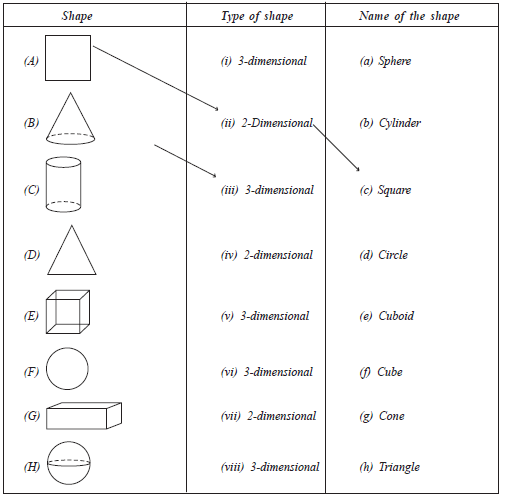Solution:
(A)      →   (ii)    →   (c)
(B)     →   (iii)    →   (g)
(C)    →   (i)      →   (b)
(D)    →   (iv)    →   (h)
(E)    →   (v)    →    (f)
(F)    →   (vii)    →  (d)
(G)    →   (vi)    →  (e)
(H)    →  (viii)    →  (a)

SOLUTION TO DO THIS
Question: Match the following pictures (objects) with their shapes:

 Picture (object) Shape (i) An agricultural field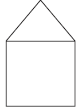(a) Two rectangular cross paths inside a rectangular park. (ii) A groove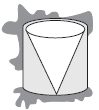(b) A circular path around a circular ground. (iii) A toy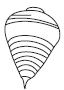(c) A triangular field adjoining a square field. (iv) A circular park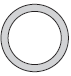(d) A cone taken out of a cylinder. (v) A cross path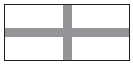(e) A hemisphere surmounted on a cone.

Solution:
(i) →  (c)
(ii) → (d)
(iii) → (e)
(iv) → (b)
(v) →  (a)

VIEW OF 3-D SHAPES
A 3-D object can look differently from different positions so it can be drawn from different perspectives.

Example 1. Let there be a combination of three cubes, one on the top of the other as shown in the adjoining figure. What will be its top and side view?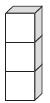Solution:
(i) If we look at this solid structure from the top, we would see just a square.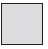(ii) If we look at it from a side, i.e. left or right, then we would see a figure as shown here.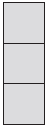Note: In this case the front view would also be the same.

Example 2. Look at the combination of four cubes as shown in the adjoining figure. Show its various views.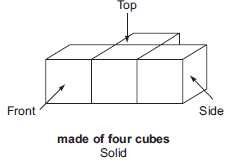Solution: The side view, front view and the top view of above solid are as given below: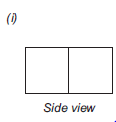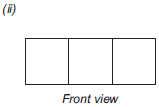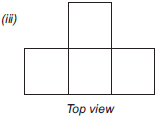EXERCISE 10.1
Question 1. For each of the given solid, the two views are given. Match for each solid the corresponding top and front views. The first one is done for you.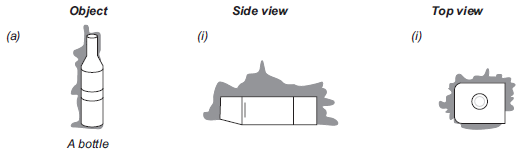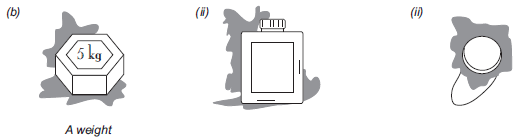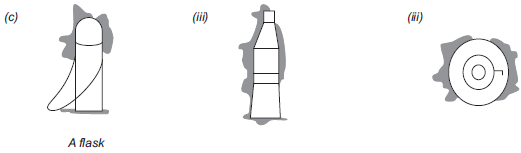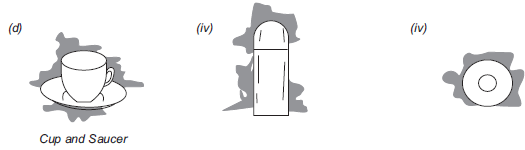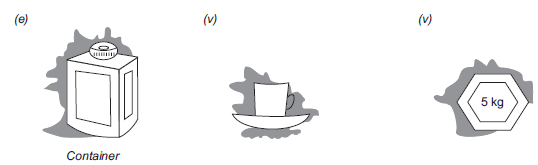Solution:
(a) → (iii) → (iv)
(b) → (i) → (v)
(c) → (iv) → (ii)
(d) → (v) → (iii)
(e) → (ii) → (i)

Question 2. For each of the given solid, the three views are given. Identify for each solid the corresponding top, front and side views.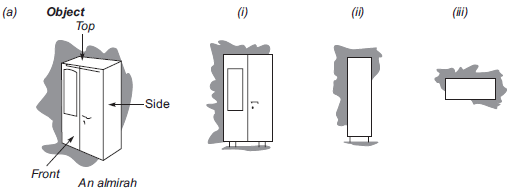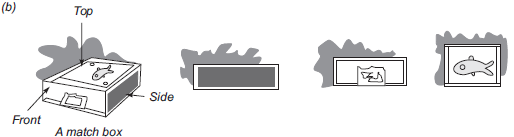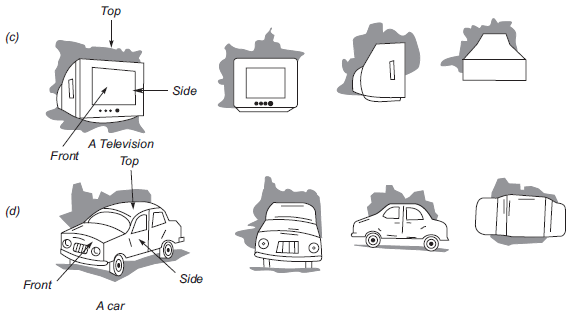Solution: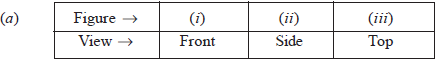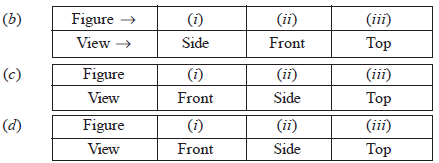Question 3. For each given solid, identify the top view, front view and side view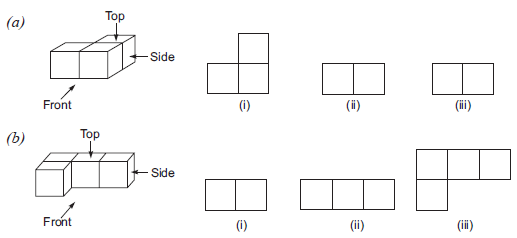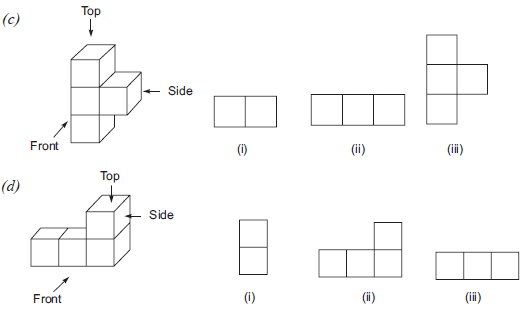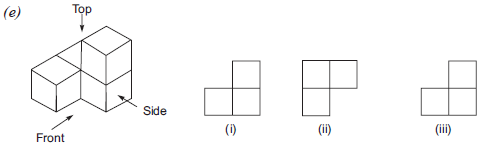Solution: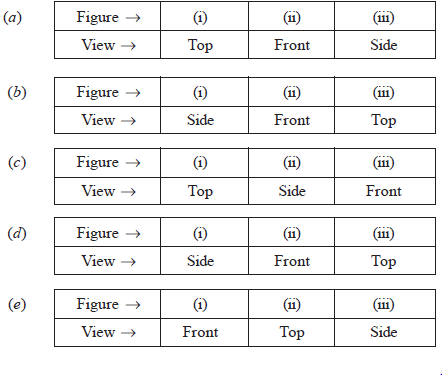Question 4. Draw the front view, side view and top view of the given objects.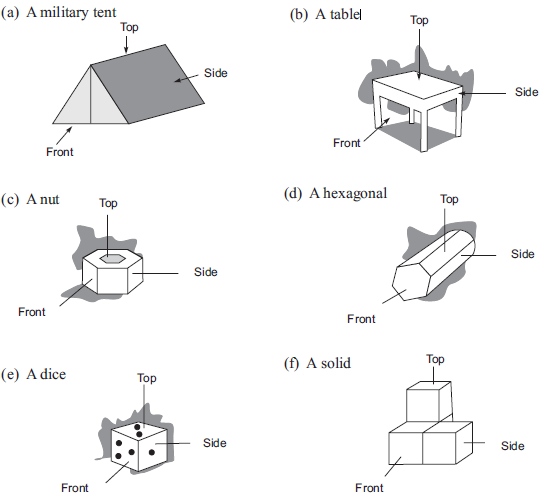Solution: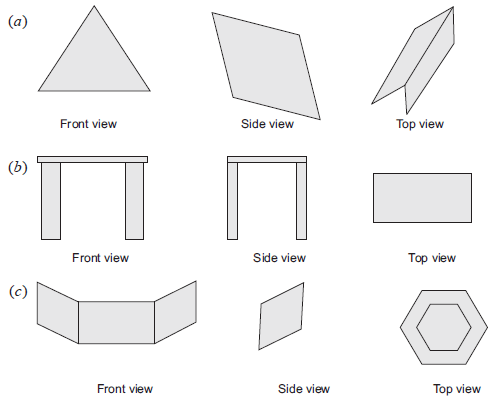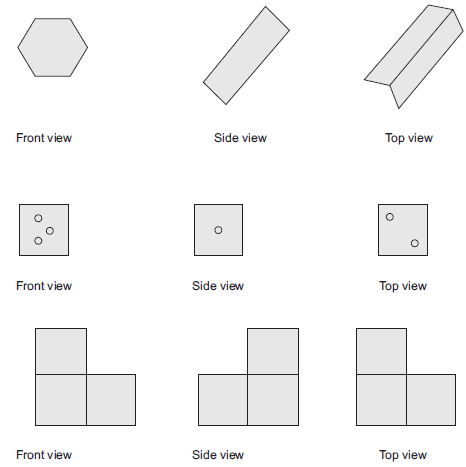Offer running on EduRev: Apply code STAYHOME200 to get INR 200 off on our premium plan EduRev Infinity!

93 docs|16 tests

,

,

,

,

,

,

,

,

,

,

,

,

,

,

,

,

,

,

,

,

,

;